# leveling

(redirected from levelings)
Also found in: Dictionary, Thesaurus.

## leveling:

see surveyingsurveying,
method of determining accurately points and lines of direction (bearings) on the earth's surface and preparing from them maps or plans. Boundaries, areas, elevations, construction lines, and geographical or artificial features are determined by the measurement of
.

## Leveling

determination of the elevations of points on the earth’s surface relative to a starting point (“zero elevation”) or to sea level. Leveling is one of the types of geodetic measurements used to create a geodetic elevation reference grid (that is, a leveling grid) and to conduct topographic surveying, as well as to plan, construct, and maintain engineering structures, railroads, highways, and so on. The results of leveling are used in research on the figure of the earth, variations in the levels of seas and oceans, and vertical movements of the earth’s crust.

Several types of leveling are distinguished, based on the method used—geometric, trigonometric, barometric, mechanical, and hydrostatic. In studying the figure of the earth, the elevations of points on the surface are determined relative to the surface of a reference ellipsoid, rather than relative to sea level, and methods of astronomical or astronomical-gravimetric leveling are used.

Geometric leveling is done by sighting on the horizontal line through the sighting tube of a level and reading the elevation of the sight line above the earth’s surface at a certain point on a rod set plumb at the point. Gradations or lines are marked on the rod. The usual method of leveling is to set the level, which is mounted on a tripod, between two rods mounted on bases or props (see Figure 1). The distances from the level to the rods depend on the required precision of leveling and on terrain conditions, but they must be approximately equal and not more than 100–150 m. The elevation h of one point above the other is determined by the difference in readings a and b from the rods, so that H = a − b. Since the points at which the rods are set are close to one another, the measured elevation of one of them relative to the other can be taken as the distance between the level surfaces passing through them.

If geometric leveling is used successively to determine the elevations of points A and B, B and C, C and D, and so on up to any remote point K, the measured elevation of point K relative to point A or the starting point O, taken as the beginning for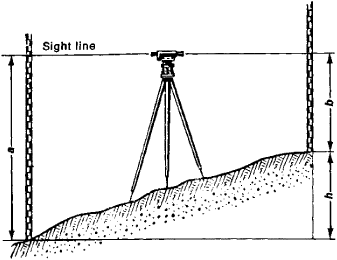Figure 1

calculating elevations, can be found by addition. The level surfaces of the earth constructed at different elevations and different points on the earth’s surface are not parallel to one another. Therefore, to determine the leveling elevation of point K it is necessary to correct the measured elevation relative to the starting point O by a factor that takes into account the nonparallel nature of the level surfaces of the earth.

The physical meaning of geometric leveling lies in the fact that the expenditure of work dW = − gdh, where g is the acceleration of gravity, is required to move a unit of mass to an infinitely small elevation dh. In terms of leveling from the starting point O to the current point K, we may write

WKWO = −(OK)gdh

where W0 and WK are the gravitational potentials at the points and the integral is computed along the path of leveling between them (the quantity obtained from this formula is called the geopotential number). Thus, leveling may be considered as a method of measuring the difference in the gravitational potentials at a given point and a starting point.

The starting point for leveling—that is, the point from which leveling elevations are figured—is taken as sea level. The leveling elevation h above sea level is determined according to the formula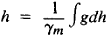where ɣm is a certain value for the acceleration of gravity; the system of leveling elevations depends on the selection of this value. A system of normal elevations measured from the average level of the Baltic Sea as determined by long-term observations relative to the zero point on a tide gauge at Kronstadt has been adopted in the USSR.

Direct leveling work is divided into classes, depending on the precision and order of the work. The State Leveling Grid of the USSR is constructed according to a special program and is divided into four classes. Class I leveling is done with high-precision leveling instruments and graduated invar rods on specially chosen lines along railroads, highways, the shores of seas and rivers, and other routes that are important for some particular reason. The mean square random error in determination of elevations on class I leveling lines is not greater than ±0.5 mm, and the systematic error is always less than ± 0.1 mm per kilometer of the route. In the USSR, class I leveling is repeated at least every 25 years, and in some areas much more often, to obtain data on possible vertical movements of the earth’s crust. The lines of class II leveling, which are laid out between the class I leveling points, form polygons with perimeters of 500–600 km and are characterized by a mean square random error of about ± 1 mm and a systematic error of ± 0.2 mm per kilometer of route. Class III and class IV leveling lines are laid out on the basis of lines of the higher classes and serve to increase the density of the leveling grid. For long-term preservation of the grid, leveling points every 5–7 km are selected and marked on the terrain with bench marks set in the ground, in the walls of stone buildings, in bridge abutments, and so on.

Trigonometric leveling, often called geodetic leveling, is based on the simple relationship of the dip angle of the sight line passing through two points on the terrain to the difference in the elevations of the points and the distance between them. A theodolite at point A is used to measure the dip angle v of a sight line that passes through the sight target at point B. If the horizontal distance s between the points and the heights of instrument / and target a are known (see Figure 2), the difference in elevations h of the points is computed according to the formula

h = s tan v + Ia

This formula is accurate only for small distances, when the curvature of the earth and the bending of a ray of light in the atmosphere may be disregarded. The more complete formula is

h = s tan v + Ia + (1 - k)s2/2R

where R is the radius of the earth as a sphere and k is the refraction coefficient.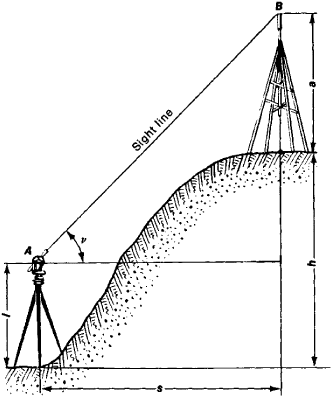Figure 2

Trigonometric leveling is used to determine the elevation of points of triangulation and traversing. It is used extensively in topographic surveying. Trigonometric leveling makes possible determination of the differences in elevation of two points that are remote from one another but are mutually optically visible. However, it is less precise than geometric leveling. The precision of the results depends primarily on the effect of the earth’s refraction, which is difficult to take into account.

Barometric leveling is based on the fact that atmospheric pressure (measured by a barometer) depends on the elevation of a point above sea level. Correction factors for the effects of temperature and air humidity are used with the measured pressure to compute elevation. Barometric leveling is common on geographic and geological expeditions, as well as for topographic surveying in inaccessible areas. Under favorable meteorological conditions the error in determining elevation is not more than 2–3 m.

Mechanical leveling is done by an automatic level, mounted on a bicycle or motor vehicle, that automatically plots the profile of the terrain and measures the distance traveled. The vertical is given by a heavy plumb in the automatic level, and the distance is recorded by a friction disk connected to the bicycle wheel. An electromechanical automatic leveling instrument is mounted on a motor vehicle and makes possible not only determination of the difference in the elevations of adjacent points and the distances between them, using appropriate counters, but also photographic analysis of the profile of the terrain.

Hydrostatic leveling is based on the fact that the free surfaces of a liquid in communicating vessels are at the same level. A hydrostatic leveling instrument consists of two glass tubes set in rods with gradations. The tubes are connected by a rubber or metal hose and are filled with a liquid, such as water or dimethyl phthalate. The difference in elevations is determined by the difference in levels of the liquid in the glass tubes; differences in temperature and pressure in different parts of the liquid of the hydrostatic level are taken into account. The error in determining the difference of elevations by this method is 1–2 mm. Hydrostatic leveling is used for continuous study of the deformation of engineering structures and for high-precision determination of the difference of the elevations of points separated by broad water obstacles.

Astronomical or astronomical-gravimetric leveling is used to determine the elevations of the geoid or quasigeoid above the reference ellipsoid. The components of deflection of the plumb in the meridian and prime vertical for each point are found by comparing the astronomical latitudes and longitudes of points on the earth’s surface with their geodetic latitudes and longitudes. Using these components, the deflections of the plumb θ on the vertical planes passing through points A and B, B and C, and so on are computed on the basis of these components, and thus the dip angles of the geoid relative to the reference ellipsoid on the planes are found. By selecting points A and B, B and C, and so on so close to one another that the change in deflections of the plumb between them can be considered linear (see Figure 3), the difference in elevations ΔΖ at adjacent points is computed according to the formula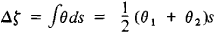If the elevation of the geoid at the starting point of leveling is known, the elevation of the geoid at any point under investigation may be found by adding the increases in elevation that have been found. By adding the elevation of the geoid to the orthometric elevation, the elevation of points on the earth’s surface above the reference ellipsoid is determined. Deflections of the plumb vary linearly from point to point only where the distances between them are small, so that astronomical leveling requires a dense grid of astronomical-geodetic points and is therefore not an advantageous method.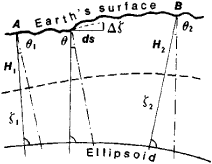Figure 3

In the USSR the effect of the nonlinear part of deflections of the plumb is taken into account according to gravimetric data. In this case astronomical leveling becomes astronomical-gravimetric leveling, which makes possible determination of the elevations of the quasigeoid and is used extensively in studies of the figure of the earth and the earth’s gravitational field.

History. Leveling originated in ancient times in connection with the construction of irrigation canals, aqueducts, and so on. The first data on a water leveling instrument are associated with the Roman architect Vitruvius (first century B.C.) and the ancient Greek scientist Hero of Alexandria (first century A.D.). Further development of leveling methods came with the invention of the sighting tube (late 16th century), the barometer (E. Torricelli, 1648), the crosshair grid in sighting tubes (J. Pi-card, 1669), and the cylindrical level (the English optician J. Ramsden, 1768).

From 1715 to 1725, I. E. Beliaev made various instruments, including a leveling instrument with a tube, in the optical workshop organized by Peter I. In the 18th century the elevations of points in Russia were determined by barometer, but the trigonometric method was used beginning in the early 19th century. The difference in the levels of the Sea of Azov and the Black Sea and the elevation of Mount Elbrus were determined by trigonometric leveling in 1836–37 under the direction of V. Ia. Struve. The direct leveling method was first used extensively in 1847 during engineering surveying for the Suez Canal. The first applications of geometric leveling in Russia during the 19th century were also related to the construction of water and land transportation routes.

In 1871 the Military Topography Department of the Main Headquarters of Russia began work to create a leveling grid for the country, and in 1913 it undertook high-precision leveling. Through their research, the Russian geodesists S. D. Ryl’ke, N. Ia. Tsinger, and I. I. Pomerantsev made major contributions to the development of the theories and methods of leveling work.

Leveling work has developed intensively in the USSR in connection with the solution of various problems of engineering and the national economy. The rates of present-day vertical movement of the earth’s crust within the entire European part of the USSR have been determined from the results of repeated leveling. The Central Scientific Research Institute of Geodesy, Aerial Photography, and Cartography has made extensive studies of the theoretical and methodological problems of leveling, which is one of the primary and most important types of present-day geodetic work.

### REFERENCES

Krasovskii, F. N. , and V. V. Danilov. Rukovodstvo po vysshei geodezii, part 1, fasc. 2. Moscow, 1939.
Zakatov, P. S. Kurs vysshei geodezii, 3rd ed. Moscow, 1964.
Chebotarev, A. S. Geodeziia, 2nd ed., parts 1–2. Moscow, 1955–62.
Eremeev, V. F. , and M. I. Iurkina. Teoriia vysot v gravitatsionnom pole Zemli. Moscow, 1972.
Izotov, A. A. , and L. P. Pellinen. Issledovaniia zemnoi refraktsii i metodov geodezicheskogo nivelirovaniia. Moscow, 1955. (7>. Tsentral’nogo n.-L in-ta geodezii, aeros”emki i kartografii, fasc. 102.)
Entin, 1.1. Vysokotochnoe nivelirovanie. Moscow, 1956. (Ibid., fasc. 111.)
Prikhoda, A. G. Barometricheskoe nivelirovanie, 2nd ed. Moscow, 1972.

A. A. IZOTOV and A. V. BUTKEVICH

## leveling

[′lev·ə·liŋ]
(engineering)
Adjusting any device, such as a launcher, gun mount, or sighting equipment, so that all horizontal or vertical angles will be measured in the true horizontal and vertical planes.
(industrial engineering)
A method of performance rating which seeks to rate the principal factors that cause the speed of motions rather than speed itself; it considers that the level at which the operator works is influenced by effort and skill.
(metallurgy)
Flattening rolled sheet by evening out irregularities, using a roller or tensile straining.
(mining engineering)
Measurement of rises and falls, heights, and contour lines. Also known as gallery.

## leveling

1. In paints, see flow, 3.
2. A surveying procedure of determining the difference in elevation between two points by means of a level or transit and a leveling rod. A spirit level is used on the level or transit to establish a horizontal line of sight.
References in periodicals archive ?
This step allowed for identification of pairs: leveling point--GNSS station in other words: pseudo-nodal points in both networks.
The distance between leveling network nodal points and GNSS permanent stations is not a constant value.
Based on the analysis, points from double leveling network and from GNSS stations (ASG EUPOS) network that are within the distance not exceeding 10 km, can be considered as corresponding to the same nodal point (pseudo-nodal point).
As mentioned, for analysis the database of four precise leveling campaigns in Poland (Kowalczyk et al., 2011) and ASG EUPOS (GNSS) stations locations were used.
The data about leveling points were obtained from the database of four precise leveling campaigns in Poland (Kowalczyk et al., 2011) previously created by the authors on the basis of many various sources, among of which analog paper catalogs and datasheets were.
First, the distances from each GNSS station to the nearest leveling point were calculated and saved as additional attributes in "GNSS stations" layer:
After the land leveling by heavy carrier-type scraper powered with rubber tires, a 2.9% uniform slope from north to south, and 0.29% uniform slope from west to east at the study area were obtained.
Before the leveling, the 30 grid centers (20 m apart) at the study area were setup with stakes by using a theodolite, level rods, and steel tapes in the mid of November 2010.
The surface subsurface soil properties (BD, sand, silt, and clay contents, pH, EC, FC, PWP, and WHC) belonging to the study area just before and after land leveling were reported as mean, standard error (SE), coefficient of variation (CV), and variance.
Paired f-tests were performed to determine whether differences in the soil properties before and after land leveling are significant or not.
The effects of land leveling on the spatial variability of the studied soil properties were determined by geostatistical analyses.
The effects of land leveling on the spatial distributions and variability of soil properties (BD, sand, silt, clay contents, pH, EC, FC, PWP, and WHC) were analyzed by mapping pre- and post-leveling values of these properties for both surface and subsurface soils.

Site: Follow: Share:
Open / Close